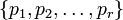Hall's theorem

Statement

Suppose$G$ is a finite group such that for any subset$\pi$ of the set of prime divisors of$G$,$G$ has a$\pi$-Hall subgroup. Then,$G$ is a Solvable group (?) (specifically,$G$ is a Finite solvable group (?)).

Facts used

1. Order has only two prime factors implies solvable (this result is popularly called Burnside's$p^aq^b$ theorem).
2. Three solvable subgroups of pairwise coprime indices implies solvable

Proof

Case that the order has one or two prime factors

If the order has two or fewer prime factors, fact (1) tells us that the group is solvable. (If the order has only one prime factor, the group is in fact nilpotent).

Case that the order has three or more prime factor

We prove this claim by induction on the number of prime factors of the order. Note that the cases of one or two prime factors have already been dealt with.

Suppose the order of$G$ is$p_1^{k_1}p_2^{k_2} \dots p_r^{k_r}$ where$p_i$ are distinct primes.

1. There exist$p_i$-complements for each prime$p_i$: In other words, for each$p_i$, there exists a subgroup$A_i$ whose index is$p_i^{k_i}$. This follows from the assumption that there exist Hall subgroups of all possible orders.
2. For any subset$\pi$ of$\{ p_1, p_2, \dots, p_r \}$, the intersection of the subgroups$A_i$ with$p_i \in \pi$, is a$\pi'$-Hall subgroup: PLACEHOLDER FOR INFORMATION TO BE FILLED IN: [SHOW MORE]
3. Each$A_i$ satisfies the hypothesis of containing Hall subgroups of all possible orders: This follows from step (2).
4.$A_i$ is solvable for each$i$: This follows from the induction hypothesis.
5.$G$ is solvable: This follows from fact (2), and the observation that when$r \ge 3$, we have a collection of at least three solvable subgroups of pairwise coprime indices.